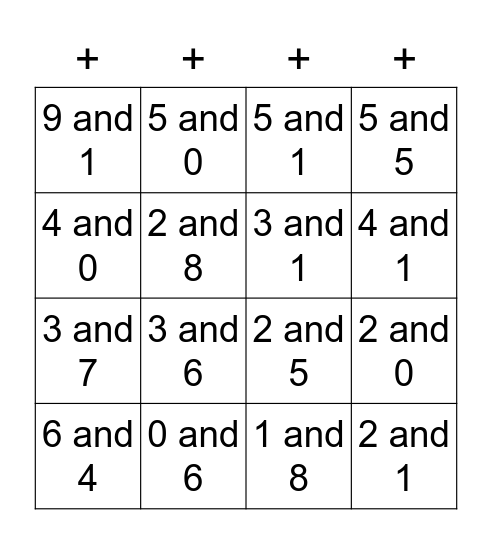This bingo card has 32 words: 0 and 1, 1 and 1, 2 and 0, 3 and 0, 2 and 1, 3 and 1, 4 and 0, 2 and 2, 5 and 0, 2 and 3, 4 and 1, 5 and 1, 3 and 3, 4 and 2, 0 and 6, 6 and 1, 3 and 4, 2 and 5, 2 and 6, 1 and 7, 4 and 4, 5 and 4, 1 and 8, 9 and 0, 6 and 4, 5 and 5, 3 and 7, 2 and 8, 9 and 1, 10 and 0, 3 and 5 and 3 and 6.

## Play Online